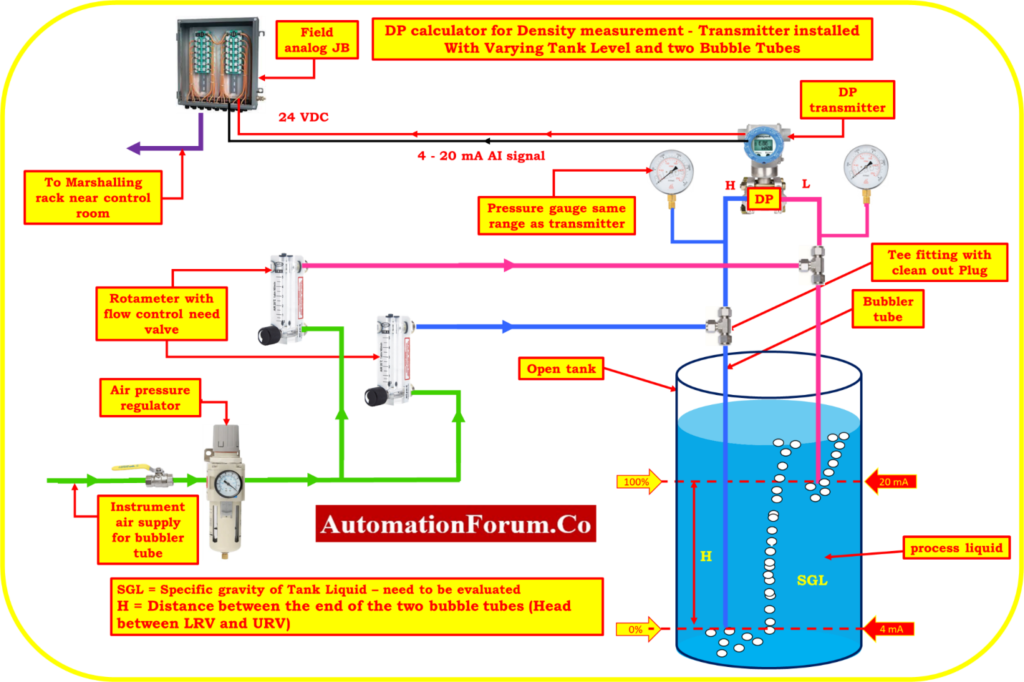# DP calculator for Density measurement – Transmitter installed With Varying Tank Level and two Bubble TubesA DP (differential pressure) calculator is used to measure density in various applications, such as in tanks with varying levels. In this particular scenario, the DP transmitter is installed with two bubble tubes in a tank with varying level.

The DP calculator uses the principle of differential pressure to determine the density of the liquid in the tank. The two bubble tubes installed in the tank are used to ensure that the pressure measurement is taken at two different levels within the tank. By measuring the difference in pressure between the two levels, the DP calculator can accurately determine the density of the liquid in the tank.

The level in the tank can vary, but the bubble tubes on both sides ensure that the pressure measurement is always taken at the same two levels within the tank. This ensures that the density measurement is accurate, even as the level of liquid in the tank changes.

Overall, a DP calculator with bubble tubes is a reliable and accurate way to measure density in tanks with varying levels. It is a useful tool in a range of industries, from oil and gas to chemical processing.

H = Distance between the end of the two bubble tubes (Head between LRV and URV)

SGL = Specific gravity of the liquid – need to be evaluated

SG1 = Minimum Specific gravity of the liquid

SG2 = Maximum Specific gravity of the liquid

H = Head between LRV and URV

Calibrated Range of the transmitter = LRV to URV

Where:

HW = Equivalent Head level pressure of Water

## Formula for Span value calculation

Span value of the transmitter = (H)*(SG2 – SG1)

## Formula for LRV estimation

In order to determine the DP, it is necessary to assume that the tank was filled to the minimum specific gravity required for the LRV calculation.

Equivalent Head level pressure of Water (HW) at Lower range value

HW at LRV = (H*SG1) = Suppression

## Formula for URV estimation

In order to determine the DP, it is necessary to assume that the tank was filled to the maximum specific gravity required for the URV calculation.

Equivalent Head level pressure of Water (HW) at Higher range value

HW at URV = (H*SG2)

## DP calculator

This below calculator used to calculate the Span value, LRV and URV of the transmitter for calibration in process liquid density measurement with varying tank level.